+1-415-670-9189
info@expertsmind.com

# Get Solution

Mechanical engineering questions
Course:- Mechanical Engineering
Reference No.:- EM13243

 TweetExpertsmind Rated 4.9 / 5 based on 47215 reviews.
Review Site
Assignment Help >> Mechanical Engineering

1. Compare liquids and gases with regard to their:

• shape

• volume occupied

• density

• viscosity

• compressibility.

2. (a) (i) Define dynamic viscosity and give the mathematical formula for Newton's law of viscosity.

(ii) How does kinematic viscosity differ from dynamic viscosity?

(b) Explain why viscosity is an important property in Fluid Mechanics.

(c) Name three classes of non-Newtonian fluids and explain how their viscosity is affected by factors other than temperature.
(d) What is the main difference between an ‘ideal' fluid and a ‘real' fluid?

3. (a) Often the mathematics of a problem in Fluid Mechanics is made easier if we assume the mass or area to be concentrated at a single point. What are these points known as for:

(i) mass

(ii) area?

(b) (i) Determine the centroid of the following shape (note that the circles are holes):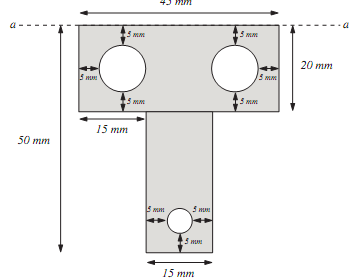(ii) Determine the second moment of area of this shape and hence its radius of gyration about the axis a-a.

4. (a) What is the formula for calculating the pressure due to the height of liquid?

(b) Name two devices which make use of this formula when used for pressure measurement.

(c) (i) In the situation, below calculate the pressure difference between points 1 and 2 for the flow of a gas within the pipe. The liquid present is mercury whose density is 13 560 kg m-3.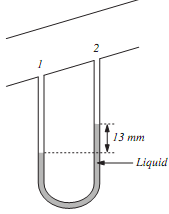(ii) The liquid is now replaced by a new liquid whose density is 2100 kg m-3. If the pressure difference remains constant, what will be the new difference in level between each limb?

(iii) What is the advantage of changing the liquid?

5. A concrete vessel containing water has a square seal gate, hinged at the top, blocking off the outflow of a large reservoir as shown below.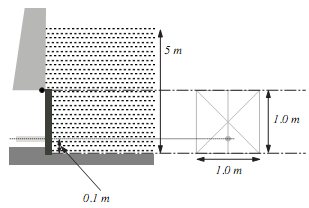(i) Calculate the total moment about the hinge of the seal gate.

(ii) To allow the water out, a circular hydraulic ram of area 0.04 m2 is to be used to open the hatch. The centre of the ram is situated 0.1 m from the bottom centre of the seal gate. Calculate the minimum pressure required on the ram to open the gate. (Assume the weight of the gate is negligible.)

6. A storage tank has the cross-sectional shape shown below and is of 1 m breadth. Calculate the resultant force acting on the inclined surface AB and its point of action. The density of the liquid is 900 kg m-3 and take g = 9.81 ms-2.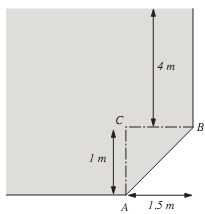7. (a) Describe the operation of a device which uses hydraulic pressure.

(b) Define:

(i) force ratio

(ii) movement ratio.

(c) It is required to lift a skip whose load total is 14 kN. Two simple lifting jacks are available both having an effort piston diameter of 10 mm and a load piston diameter of 70 mm and the load is to be distributed equally between the two. Calculate:

(i) the force ratio and movement ratio of each jack

(ii) the pressure within the hydraulic fluid.

8. The diagram shown below represents a process for which a pump and associated pipe work require to be correctly sized.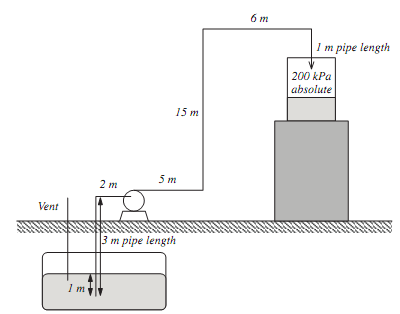The liquid is to be pumped from the underground storage vessel which is vented to atmosphere (assume 1 bar pressure) to a pressurised container supported some distance above ground level. The pump is sited at ground level and must be capable of delivering 0.01 m3 s-1 with a maximum velocity of 1.8 m s-1.

(a) Calculate the theoretical diameter of the pipe; the nominal pipe diameter chosen from the table; and the actual average velocity in the nominal pipe.

9. It is found experimentally that the terminal velocity ut of a spherical particle in a fluid depends upon the diameter d of particle, the dynamic viscosity µ of fluid and the buoyancy weight W of the particle [given by the difference in density between the particle and the
fluid (?ρ) × gravitational acceleration (g)]. Determine the nature of the relationship between these variables.

10. (a) Water (density 1000 kg m-3) is maintained at a gauge pressure of 4 MPa in a horizontal pipe of diameter 100 mm. It is passed through a nozzle of diameter 15 mm which discharges a horizontal jet into the atmosphere. If the frictional losses reduce the theoretical discharge velocity by 6% in this process, calculate the actual velocity of the jet and its flow rate in kg s-1.

(b) This jet strikes an upward curved vane moving at 15 ms-1 in a horizontal direction away from the jet, which deflects the water
through an angle of 120º. The impact is shockless. Calculate:

(i) the magnitude and direction of the velocity of the fluid leaving the vane

(ii) the thrust on the vane in a horizontal direction

(iii) the thrust on the vane in a vertical direction

(iv) the power generated by the impact in the horizontal direction.

11. (a) Distinguish, with the aid of simple sketches where appropriate, between the construction, operation and applications of the Pelton wheel, Francis turbine and Kaplan turbine.

(b) In a Francis turbine, the supply head (H) is 20 m of water (density 1000 kg m-3). The discharge rate to atmosphere is 600 kg s-1. The external radius of the runner (R1) is 0.43 m and the internal radius (R2) is 0.20 m. The runner blades are radial at inlet and they rotate at 300 revs min-1. The blades occupy 5% of the circumferential area and are shaped to ensure that the radial velocity (u1R) is kept constant and equal to  If the shaft power is 80% of the water power, determine the:

(i) guide vane angle

(ii) blade exit angle

(iii) water power

(iv) diagram power

(v) shaft power

(vi) height of runner blade at inlet and outlet.

12. A process requires the precise control of the flow of a fluid to a reactor operating at 100 bar pressure (1 bar = 100 kPa). The flowrate may need to change to match the required reactor output. The flow should be relatively non-pulsating.

(i) Suggest a suitable pump for this duty. Give reasons for your choice.

(ii) Describe, with the aid of a suitable diagram, the construction and operation of your chosen pump.

13. It is proposed to pump 1500 m3 of a liquid (density 1100 kg m-3) each day through a total head of 10 m (including all losses) by using either a centrifugal pump or a reciprocating pump.

The centrifugal pump discharges 2.0 m3 min-1 when driven by an electric motor supplying a power of 5 kW.

The reciprocating pump has a discharge rate of 1.75 m3 min-1 when driven by an electric motor supplying 3.5 kW.

(a) Calculate the efficiency of each pump.

(b) If electricity costs 8p per kWh, select the most economical pump and determine the cost saving over 300 days of operation.

(c) State two other factors which should be considered before a final choice of pump is made.

Minimize

Ask Question & Get Answers from Experts
Browse some more (Mechanical Engineering) Materials
 An Ocean Thermal Energy Conversion (OTEC) power plant built in Hawaii in 1987 was designed to operate between the temperature limits of 86°F at the ocean surface and 41°F at a Using the implicit, finite-difference method with a time increment of 1 h, find the temperature distribution in the wall 5, 10, 50, and 100 h after introduction of the flue Gravity elevators are an old idea in science fiction and consist of an evacuated tunnel dug straight through the centre of the Earth as shown below. The idea is that the eleva Construct the phase diagram for hypothetical metals A and B, between 20°C (room temperature) and 700°C, given the following information: the maximum solubility of B in A is 4w Two force F1 and F2 act on the screw eye. Their lines of action are at an angle Î¸ apart and the magnitude of each force is F1=F2=F. determine the angle between Fr and F1 in t A steel tie bar in a structure is initially 2 m long and 25 mm diameter. What will be the change in length and diameter when carrying a tensile load of 100 kN? The modulus o A single disc clutch with both sides of the disc effective is used to transmit 10 kW power at 900 r.m.p. the axial pressure is limited to 0.085 N/mm^2. If the external diamete The three members of the truss are made of ductile steel and have identical cross-sectional areas. Determine the residual stresses in each member after the load P has been i